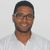# New to Qlik Sense

If you’re new to Qlik Sense, start with this Discussion Board and get up-to-speed quickly.

Announcements
Join us for a live Q&A! September 21, 10 AM ET - Onboarding Fast in Qlik Sense SaaS! REGISTER
cancel
Showing results for
Did you mean:Creator III

## How to combine two measures in qlik sense

Hi Community,

I have created two expression in qlik sense. both are working fine individually

SUM({\$<Project_Id={\$(vProjectId)}>}Budgeted_Project_Amount)

This will give total values as per our dimensions in stacked bar chart

num(SUM({\$<Project_Id={\$(vProjectId)}>}Budgeted_Project_Amount)/SUM(total <Flag_Amount>{\$<Project_Id={\$(vProjectId)}>}Budgeted_Project_Amount),'##.##%')

This will give percentages for above mentioned expression in different chart

But now i want combine these two measures i.e example if first measure value is 144 and second expression value is 28.57%

after i combine both measure i want show like this 144 (28.57%)

Thanks & Regards,

B V S S

Labels (5)

• ### Qlik Sense

3 RepliesMVP

May be :

``=SUM({\$<Project_Id={\$(vProjectId)}>}Budgeted_Project_Amount)&' ('&num(SUM({\$<Project_Id={\$(vProjectId)}>}Budgeted_Project_Amount)/SUM(total <Flag_Amount>{\$<Project_Id={\$(vProjectId)}>}Budgeted_Project_Amount),'##.##%')&')'``
Regards,
Taoufiq ZARRA

"Please LIKE posts and "Accept as Solution" if the provided solution is helpful "

(you can mark up to 3 "solutions") 😉Creator III
Author

Thank you for the response

I have tried this But it's not working

Thanks & Regards,

B V S SMVP

can you share a sample data where we can test

Regards,
Taoufiq ZARRA

"Please LIKE posts and "Accept as Solution" if the provided solution is helpful "

(you can mark up to 3 "solutions") 😉Tags
Community Browser